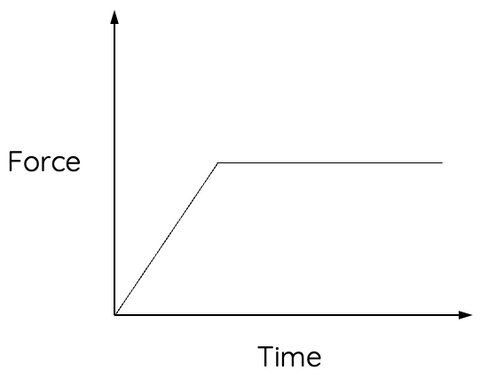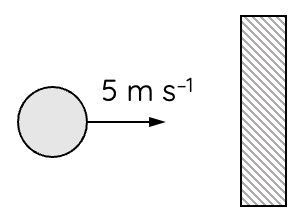# Force versus Time Graphs

This topic is part of the HSC Physics course under the section Momentum, Energy and Simple Systems.

### HSC Physics Syllabus

• evaluate the effects of forces involved in collisions and other interactions, and analyse quantitatively the interactions using the concept of impulse Δp=F_{\text{net}}Δt
• investigate the relationship and analyse information obtained from graphical representations of force as a function of time

### Force vs Time Graph, and Impulse

One of the more visually intuitive aspects of physics is the use of graphs. They provide a clear representation of abstract concepts, making it easier to comprehend the phenomena under investigation. In this article, we will specifically discuss force versus time graphs and the area under the curve (impulse), both crucial for your understanding of HSC Physics.

### Force versus Time Graphs

The force versus time graph is a graphical representation of the relationship between the force applied to an object and the time for which it is applied. The y-axis represents force (F), measured in Newtons (N), and the x-axis represents time (t), measured in seconds (s).If the force varies with time, the graph will not be a straight line. However, when the force is constant, the graph is a straight horizontal line, indicating that the force doesn't change with time.

### The Concept of Impulse

Impulse is defined as the change in momentum of an object when the object is acted upon by a force for an interval of time. It's mathematically represented as the product of force and the time period for which it acts. This leads us to the equation:

$$I = F \times t$$

where:

• I is the impulse,
• F is the force applied, and
• t is the time over which the force is applied.

The impulse provided to an object is equivalent to the change in momentum:

$$I = \Delta p$$

This statement is known as the impulse-momentum theorem. It's a powerful concept in physics, bridging the gap between force, time, and momentum.

### The Area Under the Curve

On a force versus time graph, the area under the curve represents the impulse delivered to an object. This is because the area of a rectangle is given by the product of its width (time, in this case) and height (force, in this case). Therefore, in a force-time graph, the area under the graph (force x time) gives the impulse.

Let's consider an example: if you apply a force of 10 N on an object for 5 seconds, and this is represented on a force-time graph, the area under the curve (rectangle, in this case) is 10 N x 5 s = 50 N s, which is the impulse.Even if the force changes with time (which means the graph is not a rectangle), the area under the curve still gives the total impulse. In some cases, the area under the curve can be calculated by finding the area of triangles, trapeziums etc.

Solutions to the following examples can be found in the video above.

### Example 1

A force versus time applied on a mass is shown by the graph.What is the change in momentum due to the force applied?

### Example 2

A 60 kg mass starts moving from rest due to an applied force as shown by the graph.(a) Calculate the speed of the mass at t = 5 s.

(b) Calculate the work done from t = 0 to t = 5 s.

### Example 3

A 0.2 kg ball is thrown at 5 m s–1 at a frictionless wall as shown.The magnitude of force exerted by the wall on the ball during their contact over time is shown by the graph.At what speed does the ball rebound off the wall?